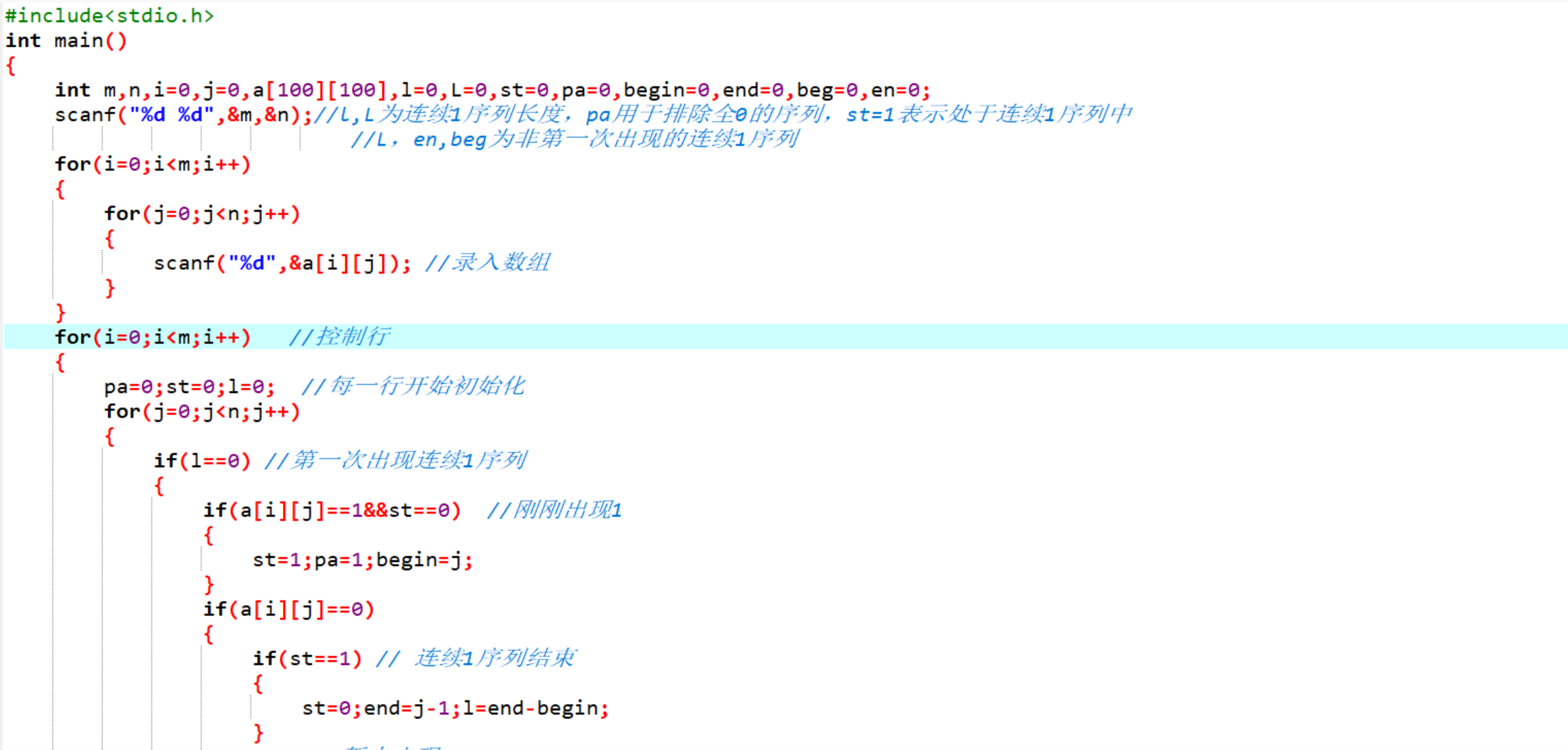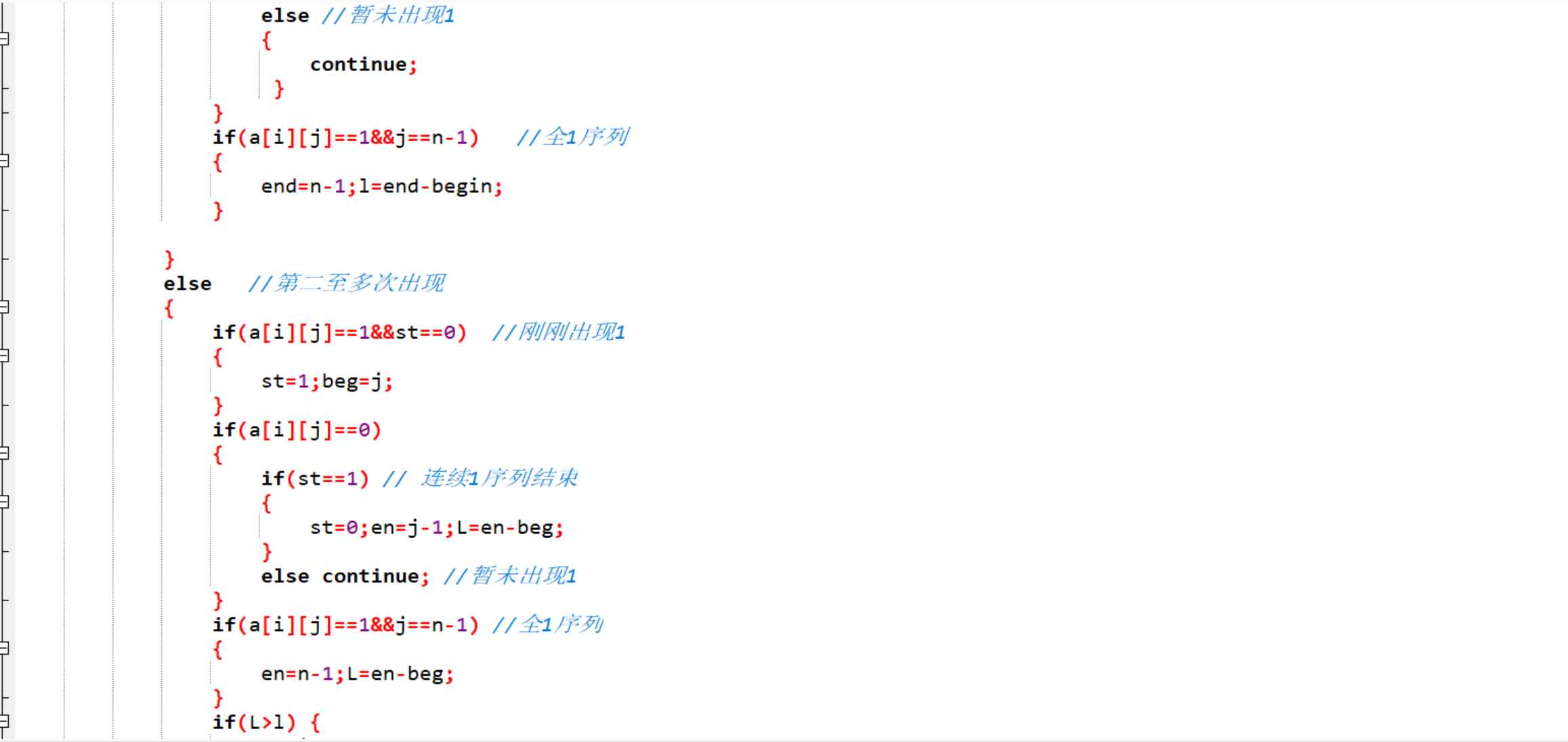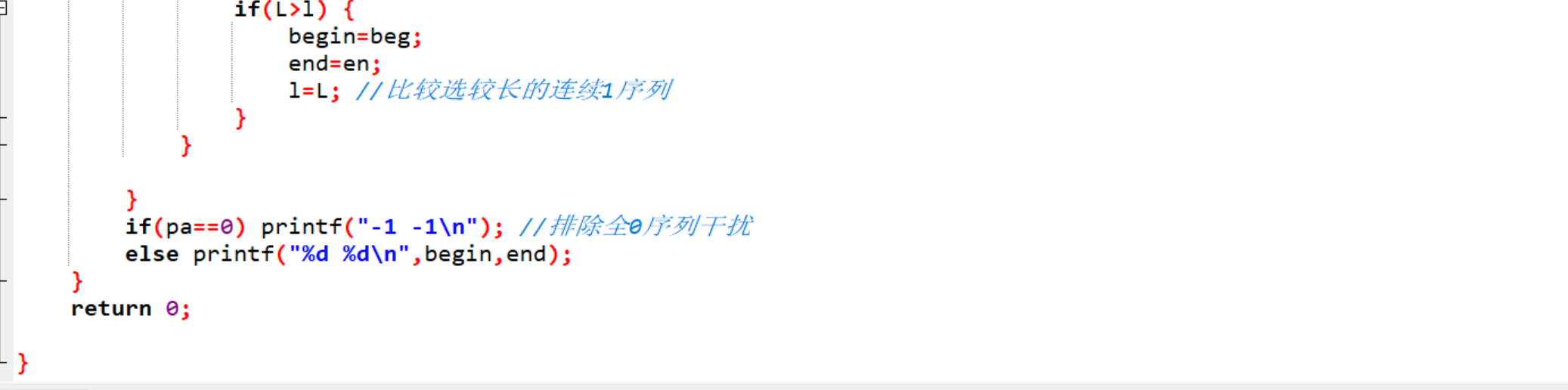m0_74851736 2023-01-11 21:01 采纳率: 80%

# 关于#c++#的问题：输入说明输入第一行为两个整数m和n(0<=m,n<=100)表示二维数组行数和列数，输入数据中不会出现同一行有两个最长1序列的情况

0-1矩阵

2S

1000Kb

5 6
1 0 0 1 1 0
0 0 0 0 0 0
1 1 1 1 1 1
1 1 1 0 1 1
0 0 1 1 0 0

3 4
-1 -1
0 5
0 2
2 3• 写回答

#### 1条回答默认 最新

•Sirius·Black 2023-01-12 15:57
关注

略有改动

``````
#include <bits/stdc++.h>
int main()
{
int m,n,i,j,ma,p,begin,Begin,End,a;
scanf ("%d%d",&m,&n);
for (i=0;i<m;i++)
for (j=0;j<n;j++)
scanf ("%d",&a[i][j]);
for (i=0;i<m;i++)
{
ma=0; p=0;
for (j=0;j<n;j++)
{
if (a[i][j]==1)
{
if (p==0)
begin=j;
p++;
}
else
{
if (ma<p)
{
ma=p;
Begin=begin;
End=begin+p-1;
}
p=0;
}
}
if (ma<p)
{
ma=p;
Begin=begin;
End=begin+p-1;
}
if (ma==0)
printf ("-1 -1\n");
else
printf ("%d %d\n",Begin,End);
}
return 0;
}
``````
本回答被题主选为最佳回答 , 对您是否有帮助呢?
评论

#### 悬赏问题

• ¥40 找同学帮敲Python代码
• ¥15 MYSQL 订单的商品明细重复计算问题
• ¥15 微信实时共享位置修改
• ¥100 TG的session协议号转成直登号号后客户端登录几分钟后自动退出设备
• ¥50 共模反馈回路的小信号增益
• ¥15 arduino ssd1306函数与tone函数放歌代码不兼容问题
• ¥70 0.96版本hbase的row_key里含有双引号，无法deleteall
• ¥40 Ida Pro增加插件出现问题
• ¥15 诊断性META分析合并效能的检验
• ¥15 请问abb根据色块判断奇偶数并根据批次号放入仓储